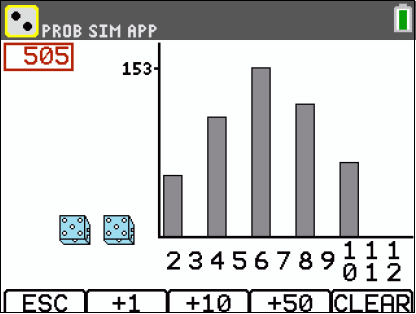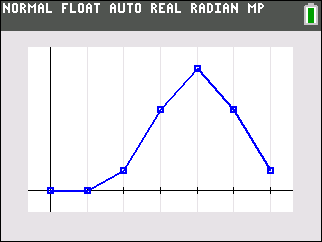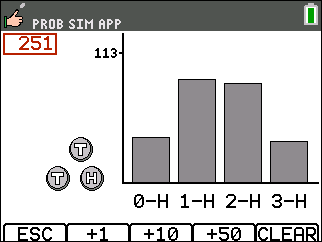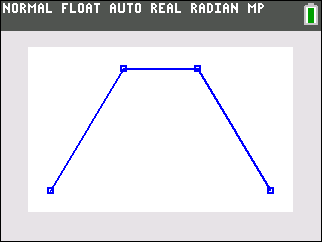# Activities

•• ##### Author45 Minutes

## Roll the Dice#### Activity Overview

Students compute sample spaces for coin flips, find the various probabilities associated, and graph the probabilities using the calculator.

#### Key Steps

•Students will calculate the probability and graph the distribution of using unfair coins.

•Students will calculate the probability of all possible outcomes of tossing three coins.

•Students will graph the distribution.